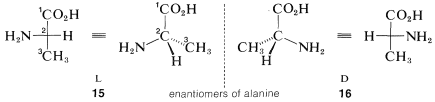# 5.5: The D, L Convention for Designating Stereochemical Configurations

$$\newcommand{\vecs}{\overset { \rightharpoonup} {\mathbf{#1}} }$$ $$\newcommand{\vecd}{\overset{-\!-\!\rightharpoonup}{\vphantom{a}\smash {#1}}}$$$$\newcommand{\id}{\mathrm{id}}$$ $$\newcommand{\Span}{\mathrm{span}}$$ $$\newcommand{\kernel}{\mathrm{null}\,}$$ $$\newcommand{\range}{\mathrm{range}\,}$$ $$\newcommand{\RealPart}{\mathrm{Re}}$$ $$\newcommand{\ImaginaryPart}{\mathrm{Im}}$$ $$\newcommand{\Argument}{\mathrm{Arg}}$$ $$\newcommand{\norm}{\| #1 \|}$$ $$\newcommand{\inner}{\langle #1, #2 \rangle}$$ $$\newcommand{\Span}{\mathrm{span}}$$ $$\newcommand{\id}{\mathrm{id}}$$ $$\newcommand{\Span}{\mathrm{span}}$$ $$\newcommand{\kernel}{\mathrm{null}\,}$$ $$\newcommand{\range}{\mathrm{range}\,}$$ $$\newcommand{\RealPart}{\mathrm{Re}}$$ $$\newcommand{\ImaginaryPart}{\mathrm{Im}}$$ $$\newcommand{\Argument}{\mathrm{Arg}}$$ $$\newcommand{\norm}{\| #1 \|}$$ $$\newcommand{\inner}{\langle #1, #2 \rangle}$$ $$\newcommand{\Span}{\mathrm{span}}$$$$\newcommand{\AA}{\unicode[.8,0]{x212B}}$$

We pointed out in Chapter 3 the importance of using systematic names for compounds such that the name uniquely describes the structure. It is equally important to be able to unambiguously describe the configuration of a compound. The convention that is used to designate the configurations of chiral carbons of naturally occurring compounds is called the $$D, L$$ system. To use it, we view the molecule of interest according to the following rules:

1. The main carbon chain is oriented vertically with the lowest numbered carbon at the top. The numbering used for this purpose must follow the IUPAC rules:2. Next, the structure must be arranged at the particular chiral carbon whose configuration is to be assigned so the horizontal bonds to that carbon extend toward you and the vertical bonds extend away from you. This arrangement will be seen to be precisely the same as the convention of projection formulas such as $$5c$$ and $$6c$$ (Section 5-3C).

3. Now the relative positions of the substituents on the horizontal bonds at the chiral centers are examined. If the main substituent is the left of the main chain, the $$L$$ configuration is assigned; if this substituent is on the right, the $$D$$ configuration is assigned.

For example, the two configurations of the amino acid, alanine, would be represented in perspective or projection as $$15$$ and $$16$$. The carboxyl carbon is $$C1$$ and is placed at the top. The substituents at the chiral carbon connected to the horizontal bonds are amino ($$-NH_2$$) and hydrogen. The amino substituent is taken to be the main substituent; when this is on the left the acid has the $$L$$ configuration, and when it is on the right, the $$D$$ configuration. All of the amino acids that occur in natural proteins have been shown to have the $$L$$ configuration.Glyceraldehyde, $$CH_2OHCHOHCHO$$, which has one chiral carbon bonded to an aldehyde function, hydrogen, hydroxyl, and hydroxymethyl ($$CH_2OH$$), is of special interest as the simplest chiral prototype of sugars (carbohydrates). Perspective views and Fischer projections of the $$D$$ and $$L$$ forms correspond to $$17$$ and $$18$$, respectively, where the carbon of the aldehyde function ($$-CH=O$$) is $$C1$$:The $$D,L$$ system of designating configuration only can be applied when there is a main chain, and when we can make an unambiguous choice of the main substituent groups. Try, for instance, to assign $$D$$ and $$L$$ configurations to enantiomers of bromochlorofluoromethane. An excellent set of rules has been worked out for such cases that leads to unambiguous configurational assignments by what is called the $$R,S$$ convention. We discuss the $$R,S$$ system in detail in Chapter 19 and, if you wish, you can turn to it now. However, for the next several chapters, assigning configurations is much less important to us than knowing what kinds of stereoisomers are possible.